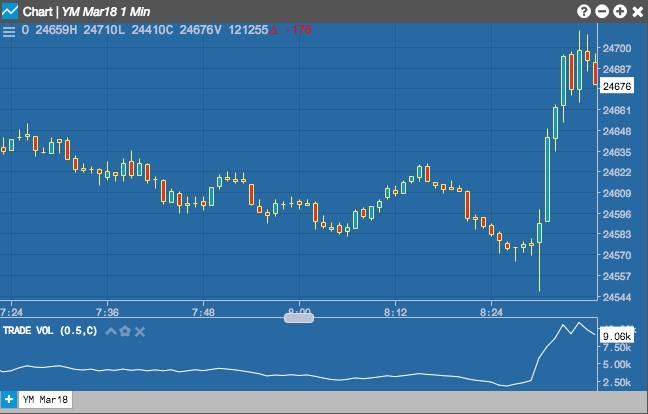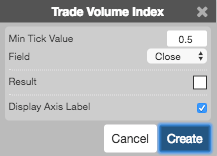# Charts

Charts

The Trade Volume Index (TVI) leverages intraday market data to show whether a security is being accumulated (purchased) or distributed (sold). The Trade Volume Index assumes that higher prices represent buy orders while lower prices are sell orders.## Configuration Options• Min Tick Value: Minimum price movement required to include in the calculation.
• Field: Price or combination of prices to use as the base for average calculations. Possible values include:
• Open
• High
• Low
• Close
• HL/2 $$\left ( \frac{High + Low}{2} \right )$$
• HLC/3 $$\left ( \frac{High + Low + Close}{3} \right )$$
• HLCC/4 $$\left ( \frac{High + Low + Close + Close}{4} \right )$$
• OHLC/4 $$\left ( \frac{Open + High + Low + Close}{4} \right )$$
• Color Selectors: Colors to use for graph elements.
• Display Axis Label: Whether to display the most recent value on the Y axis.

## Formula

$EMA1 = EMA1_\text{n-1} + \left ((\frac{2}{(n + 1)}) * (P_n - EMA1_\text{n-1}) \right)$

$EMA2 = EMA2_\text{n-1} + \left ((\frac{2}{(n + 1)}) * (EMA1_n - EMA2_\text{n-1}) \right)$

$EMA3 = EMA3_\text{n-1} + \left ((\frac{2}{(n + 1)}) * (EMA2_n - EMA3_\text{n-1}) \right)$

$TRIX = \frac{(EMA3_n - EMA3_\text{n-1})}{EMA3_\text{n-1}}$

where:

• Pn =the current price.
• EMA1n-1 = the exponential moving average value of n periods back
• EMA2n-1 = the exponential moving average value of n periods back
• EMA3n-1 = the exponential moving average value of n periods back